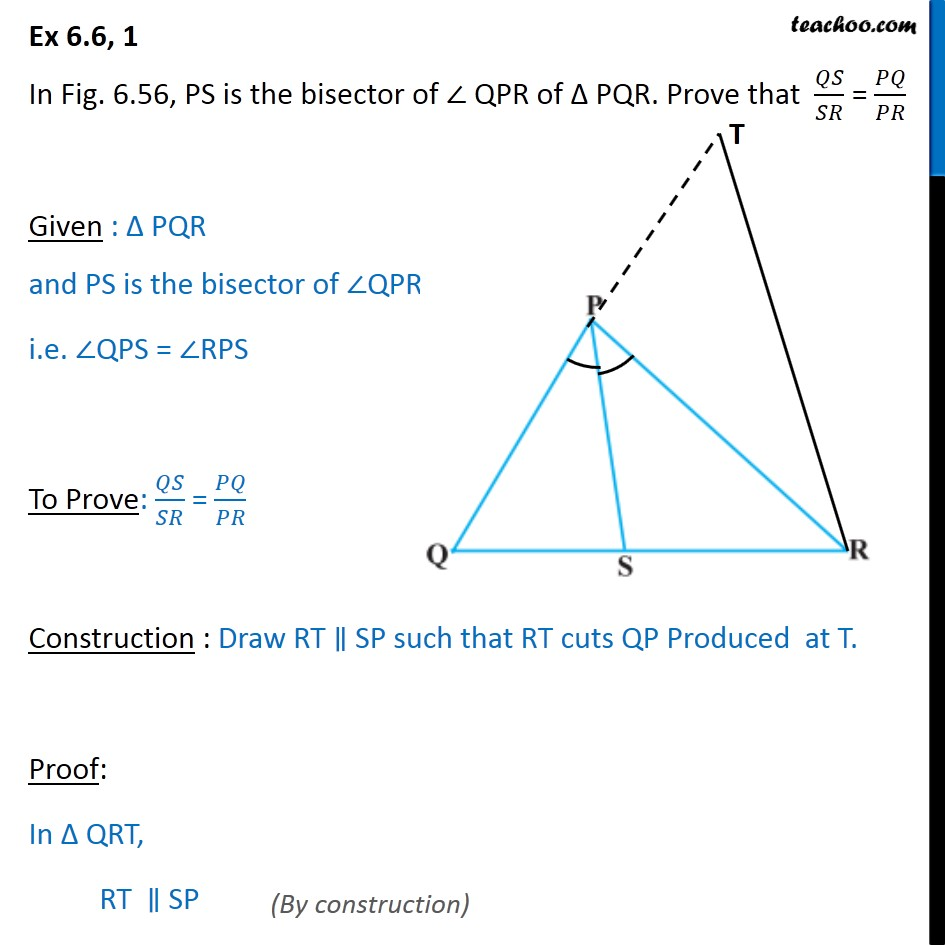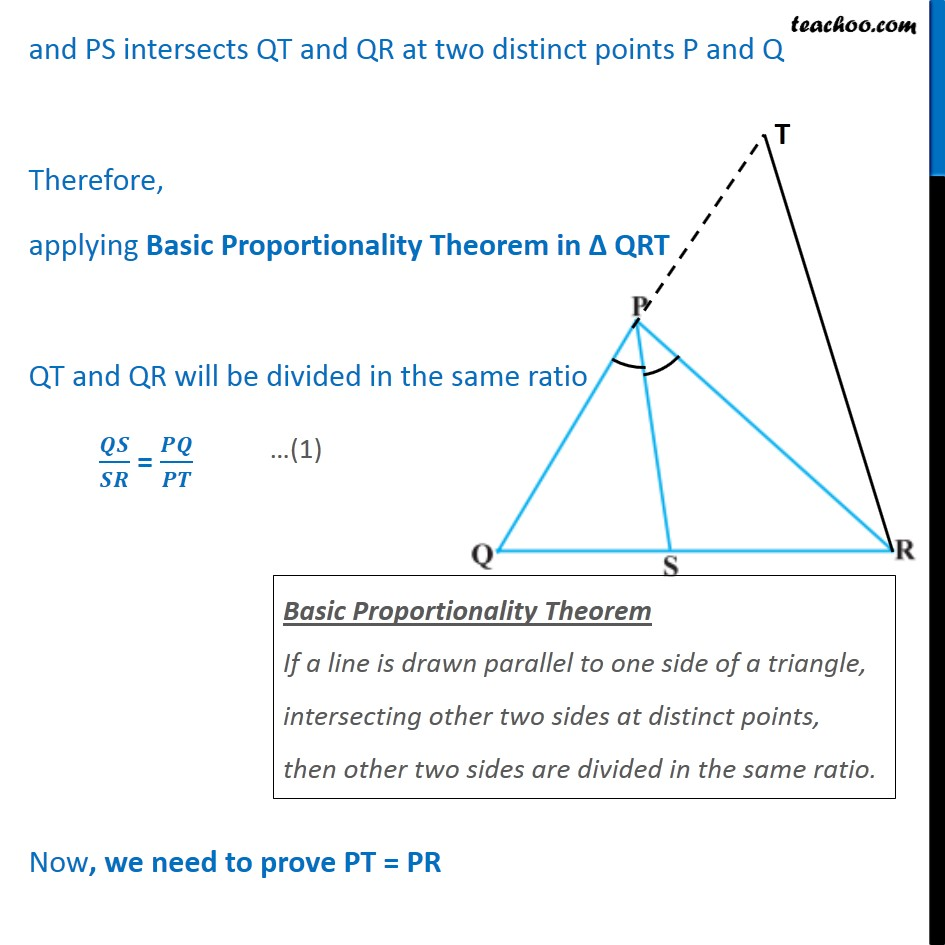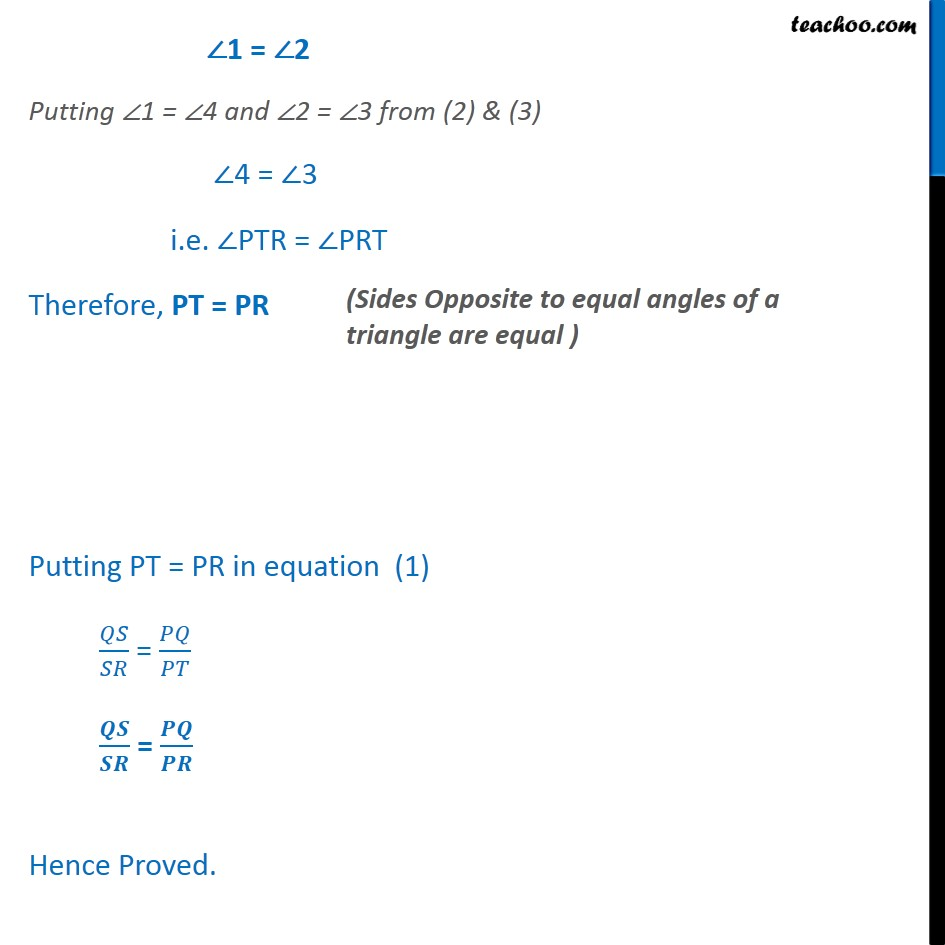Important questions of Triangle (in Geometry)

Chapter 6 Class 10 Triangles
Serial order wiseLearn in your speed, with individual attention - Teachoo Maths 1-on-1 Class

### Transcript

Question 1 In Fig. 6.56, PS is the bisector of QPR of PQR. Prove that / = / Given : PQR and PS is the bisector of QPR i.e. QPS = RPS To Prove: / = / Construction : Draw RT SP such that RT cuts QP Produced at T. Proof: In QRT, RT SP and PS intersects QT and QR at two distinct points P and Q Therefore, applying Basic Proportionality Theorem in QRT QT and QR will be divided in the same ratio / = / Now, we need to prove PT = PR Now RT SP & PR is the transversal Therefore, Also, Given that PS is the bisector of QPR QPS = RPS 1 = 2 Putting 1 = 4 and 2 = 3 from (2) & (3) 4 = 3 i.e. PTR = PRT Therefore, PT = PR Putting PT = PR in equation (1) / = / / = / Hence Proved.